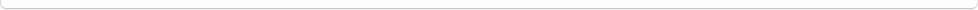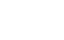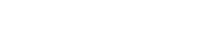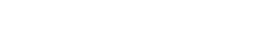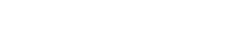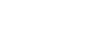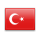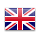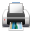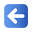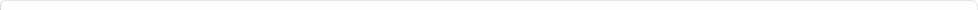Description of Individual Course Units
 Course Unit Code Course Unit Title Type of Course Unit Year of Study Semester Number of ECTS Credits 190105004105 STATISTICS Compulsory 2 4 5
Level of Course Unit
First Cycle
Objectives of the Course
The aim of this course is to enable researchers to use and apply statistical concepts and statistical tests. The course includes basic statistical concepts and the use of statistical techniques. Within the scope of the course, it is taught how to use these techniques practically in the computer environment.
Name of Lecturer(s)
Dr. Öğretim Üyesi Turgay Demirel
Learning Outcomes
 1 To know the basic concepts and methods related to statistics 2 Recognizing the importance of statistics in scientific research 3 Ability to properly read statistical representations such as graphs and tables 4 To be able to make sense of central tendency and diffusion measures 5 Being able to comment on the relevant group by interpreting certain parameters based on the characteristics of the normal distribution. 6 Knowing the rationale and steps of hypothesis testing 7 Ability to select and use appropriate tests to compare group means 8 Organizing, applying, and analyzing data to reveal relationships between values 9 To be able to perform mean comparison and correlation calculations by using parametric and non-parametric tests in SPSS program, to be able to show and interpret analysis findings appropriately.
Mode of Delivery
Daytime Class
Prerequisites and co-requisities
Recommended Optional Programme Components
-
Course Contents
Introduction to Statistics, Basic Concepts (Variables, Scales) Frequency Distributions Using Tables and Graphs, Central Tendency and Distribution Measures, Normal Distribution, Skewness and Kurtosis, Statistical Tests and General Principles, Hypothesis Test Process, t-Test, Analysis of Variance, Correlation, Non- Parametric Tests
Weekly Detailed Course Contents
 Week Theoretical Practice Laboratory 1 Introduction to Statistics Giving information about the aim, scope and resources of the course Introduction to Statistics Use of Statistics in Research Descriptive and Predictive Statistics Population, Sample SPSS Setup General introduction of SPSS 2 Basic Concepts (Variables, Scales) Frequency Distributions Using Tables and Graphs Frequency Distributions Tables and Properties Table Types Frequency Table Crosstab Graphics and Features Chart Types Bar, Pie, Histogram, Boxplot, Line, Scatter (Scatter plot) Entering data into SPSS frequency table creation Graph creation Entering data into SPSS frequency table creation Graph creation 3 Central Tendency and Distribution Measures Central Tendency Measures Mean, Median, Mode Central Distribution Measures Variance, Standard Deviation, Range, Quarter Deviation(Interquartil), Percentile Cleaning up data Re-coding Total score and average score calculation Descriptive statistics applications Cleaning up data Re-coding Total score and average score calculation Descriptive statistics applications 4 Normal Distribution, Skewness and Kurtosis Normal distribution Standard Normal Distribution (Z-Score) Distortion Kurtosis Normality Tests and reporting Kolmogorov-Smirnov Test Shapiro-Wilk Test Transforming Data Checking the normal distribution Checking the normal distribution 5 Tests and General Principles Precision Level Range Estimation Sample Distribution Central Limit Theorem Standard error Confidence Interval meaningfulness assumptions Data Entry application Data Entry application 6 Hypothesis Testing Process Hypothesizing Zero Hypothesis (Null Hypothesis) Counter-Hypothesis/Research Hypothesis (Alternative Hypothesis) Setting the Decision Rule Test Calculation One-way and Two-Way Test (Onetailed two-tailed test) Significance Value (pvalue) Type I and Type II error (Type I and Type II error) Statistical Power To decide Hypothesis testing questions 7 MIDTERM EXAM 8 t-Test What is t-test? t-test Assumptions t-test types Single sample t-Test (Onesample t-test) Reporting Making dependent and independent samples t-test on the data, interpreting it, writing the APA conclusion section Making dependent and independent samples t-test on the data, interpreting it, writing the APA conclusion section 9 Analysis of Variance What is Analysis of Variance? Analysis of Variance Assumptions Variance Homogeneity (Levene Test) Rate of Variability Types of Analysis of Variance One Way Variance analysis For Repeated Measurements One-way ANOVA Factorial ANOVA Covariance Analysis One-way ANOVA Multiple Comparison Tests (Post hoc) One-way ANOVA on the data, interpretation, writing APA result section One-way ANOVA on the data, interpretation, writing APA result section 10 correlation correlation Scatter Diagrams Correlation Value Co-Exchange Correlation Coefficients Explanatory Coefficient Ranking Data Factors Affecting Correlation Extreme Situations Correlation and Reasoning Correlation Analysis Pearson Correlation Spearman Correlation Partial Correlation Effect Size Reporting What is Regression? Correlation on data, interpretation, writing APA results section Correlation on data, interpretation, writing APA results section 11 Non-Parametric Tests Chi-square (Ki Kare) Mann-Whitney U Test Wilcoxon’s Matched-Pairs Tests Signed-Ranks Tests Kruskal-Wallis Tests Friedman’s Rank Tests Performing and interpreting non-parametric tests on data Performing and interpreting non-parametric tests on data 12 Practice Session Performing parametric and non-parametric tests on the given data
Lecture notes shared on the Course Web page IBM SPSS Statistics 20 Brief Guide ftp://public.dhe.ibm.com/software/analytics/spss/documentation/statistics/20.0/en/client/Manuals/IBM_SPSS_Statistics_Brief_Guide.pdf Field, A. (2009). Discovering statistics using SPSS (3rd edition). London: Sage Pallant, J. (2016). SPSS Kullanma Kılavuzu: SPSS ile Adım Adım Veri Analizi (Balcı, S. ve Ahi, B. Çev.) Ankara:ANI Yayıncılık
Planned Learning Activities and Teaching Methods
Assessment Methods and Criteria
 Term (or Year) Learning Activities Quantity Weight Midterm Examination 1 40 Attending Lectures 1 10 Laboratory 1 50 SUM 100 End Of Term (or Year) Learning Activities Quantity Weight Final Examination 1 50 Report Preparation 1 50 SUM 100 Term (or Year) Learning Activities 40 End Of Term (or Year) Learning Activities 60 SUM 100
Language of Instruction
Work Placement(s)
-